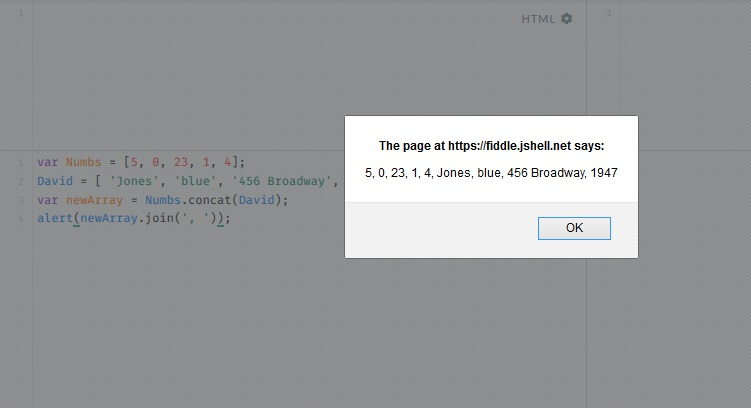# Learn About Arrays in JavaScript

0
2638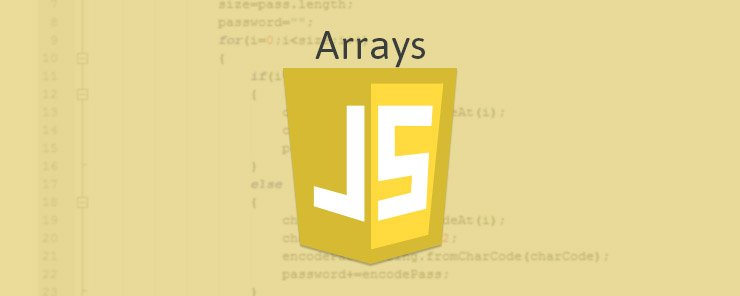This tutorial is going to revolve around JavaScript arrays. An array can store a multitude of different values of different data types. It is not required to define a size for a JavaScript array.

Let’s construct an example array. David’s Last Name is Jones, his eyes are blue, and he lives on 456 Broadway, and was born in 1947.

`David = [ 'Jones', 'blue', '456 Broadway', 1947];`

Every value in an array has an index, starting from an index of 0 for the first value. In our David array:

 Jones blue 456 Broadway 1947 0 1 2 3

We can use the index to access and output values from the array, like so:

`document.write( 'second index:' + David1 + '</br>');`

It’s rather simple to add values to an array using the index. For example, if we wanted to add a hair color value to our David array at the 5th position, we could write:

`David = 'blond';`

You can also override values in an array. For example, if we want to override only one value, for example, of index 2, we can use the splice() method.

`David.splice(2, 1, 'NY');`

This basically means “splice 1 value(s) starting at index 2”.  And the value ‘456 Broadway’ will be replaced with ‘NY’.

We can also override and add values to the array at the same time, like so:

`David.splice(2, 1, 'NY', 'blond');`

This would replace the value at index 2 with ‘NY’, add the value ‘blond’ afterwards, and “push” the remaining array values one step forward.
The result of the above would be:

 Jones blue NY blond 1947 0 1 2 3 4

We can also use splice() to delete values in the array, like so:
ArrayName.splice(index, number of values);

For example, if we want to delete the first value in our array, we would write:

`David.splice(0,1);`

Another way to delete the value in index 0 would be:

`delete David;`

We can convert our array into a string in several different ways:

1. With the valueOf() function, like so:
`David.valueOf();`
1. With the join() function, like so:
`David.join(', ');`

In the latter, we are adding a comma and a space between each two values, like so: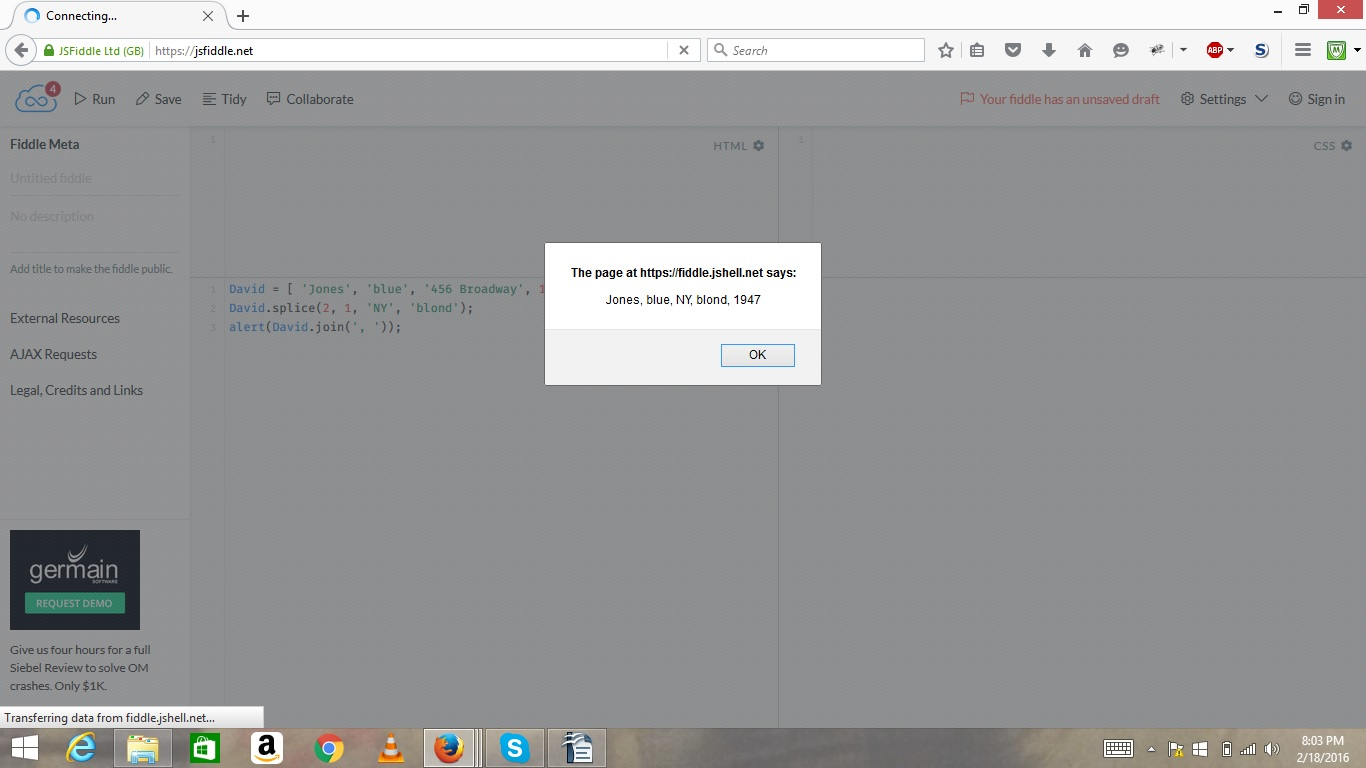1. With the toString() function, like so:
`David.toString();`

We can remove the last value from an array with pop(), like so:

`David.pop();`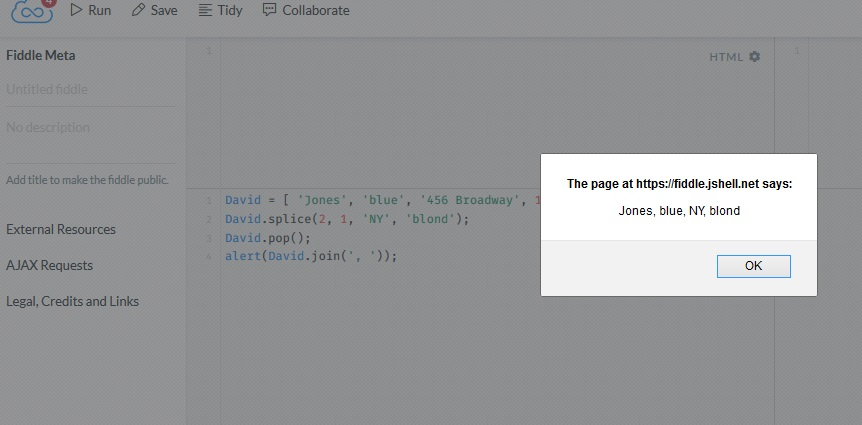We can add values to the end of an array (no overriding), using push(), like so:

`David.push('new value 1', 'new value 2');`

These 2 values would be pushed to the end of the David array.We can delete the first item in our array, using shift(), like so:

`David.shift();`

We can add items to the very beginning of the array with unshift(), like so:

`David.unshift('bowie');`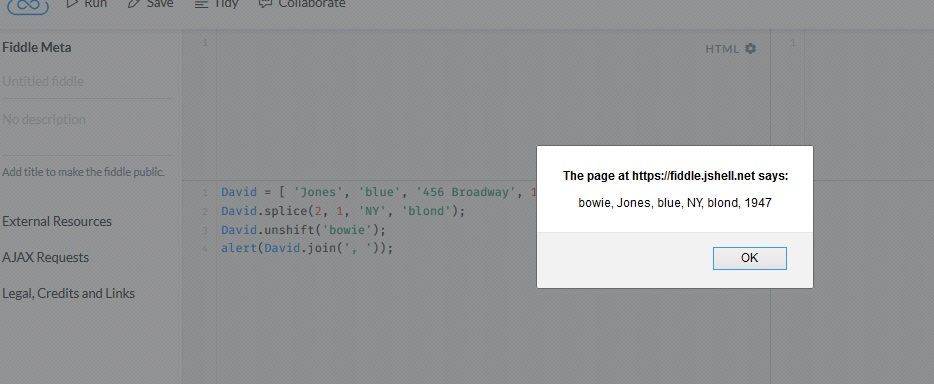We can iterate over the array using a for loop and a variable that gets incremented as long as it’s smaller than the length of the array, which we can get with ArrayName.length. Let’s use this loop to print out our current array:

```for(i=0; i<David.length; i++){
document.write(David[i] + '<br/>');
}
```

If we have an array of numbers, we can sort the array with the sort() function. Example:

`var Numbs = [5, 0, 23, 1, 4];`

To sort the above array in ascending order, we would write:

`Numbs.sort(function(x,y){return(x-y)});`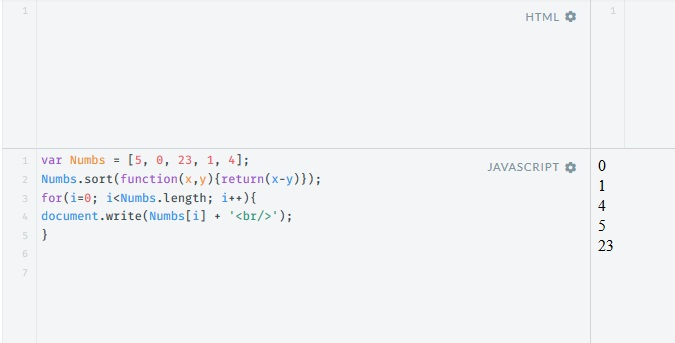To sort the above array in descending order, we would write:

`Numbs.sort(function(x,y){return(y-x)});`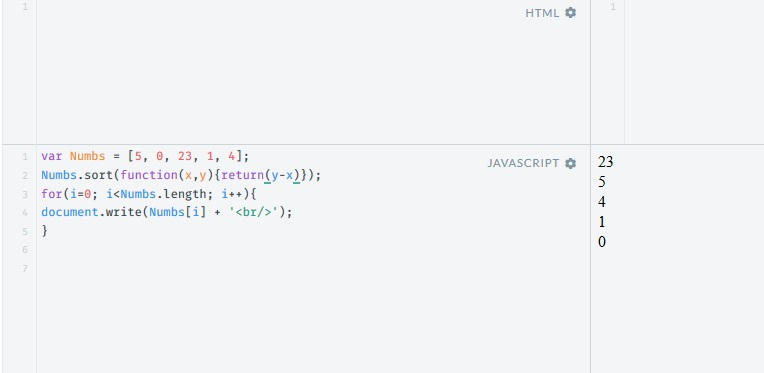You can combine several arrays into one arrays using the concat() function, like so:

`var newArray = Numbs.concat(David);`

We’re going to turn the resulting array into a string using join() and alert the result, like so:

`alert(newArray.join(' , ')):`

Below is the output you will get.